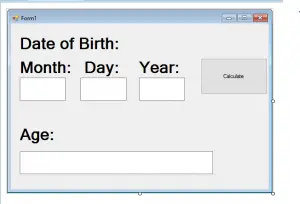# How to Calculate Age using VB.Net

0
1676

This tutorial is all about How to Calculate Age using VB.Net. With this tutorial you can Calculate Age using VB.Net. easily. So let’s get started:

• First is open the Visual Basic, Select File on the menu, then click New and create a new project.• Then a New Project Dialog will appear. You can rename your project, depending on what you like to name it. After that click OK• Design your form like this just like what I’ve shown you below.
Add a Label, 4 Textbox, and a Button.[vbnet]
Private Sub Button1_Click(ByVal sender As System.Object, ByVal e As System.EventArgs) Handles Button1.Click
Dim a As String
Dim b As String
Dim c As String
a = TextBox3.Text
b = TextBox1.Text
c = TextBox2.Text
Dim DOB As New DateTime(a, b, c)
Dim Years As Integer = DateDiff(DateInterval.Year, DOB, Now) – 1
Dim Months As Integer = DateDiff(DateInterval.Month, DOB, Now) Mod 12
Dim days As Integer = DateDiff(DateInterval.Day, DOB, Now) Mod 30 – 10
TextBox4.Text = Years & ” Years, ” & Months & ” Months ”
End Sub
[/vbnet]

• Finally, Click F5 to run Program.
Output: